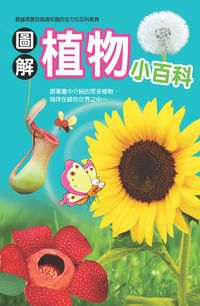### 圖解植物小百科

• 點閱：1
• 其他題名：植物小百科
• 作者：
• 出版年：2013[民102]
• 出版社：幼福文化
• 出版地：新北市
• 集叢名：兒童知識通
• ISBN：978-957-747-813-9 ; 957-747-813-1
• 附註：主要內容國語注音

●各形各態的花草植物
●植物分類的特點說明
●豐富精美的彩色圖片
●趣味的植物冒險漫畫

##章節

• 前言(p.4)
• 植物大家庭(p.10)
• 向日葵成長日記(p.12)
• 綠藻(p.14)
• 海帶(p.16)
• 墨角藻(p.18)
• 紫菜(p.20)
• 地錢(p.22)
• 鹿角蕨(p.24)
• 鳳尾蕨(p.26)
• 毛地黃(p.28)
• 罌粟(p.30)
• 植物冒險漫畫 ❶ 夢想中的花園(p.32)
• 銀杏(p.36)
• 水杉(p.38)
• 紅豆杉(p.40)
• 側柏(p.42)
• 榕樹(p.44)
• 冷杉(p.46)
• 槐樹(p.48)
• 松樹(p.50)
• 椰子樹(p.52)
• 竹子(p.54)
• 蘆葦(p.56)
• 海芋(p.58)
• 火鶴花(p.60)
• 天堂鳥(p.62)
• 蘆薈(p.64)
• 百合(p.66)
• 鬱金香(p.68)
• 鈴蘭(p.70)
• 拖鞋蘭(p.72)
• 鳶尾花(p.74)
• 唐菖蒲(p.76)
• 水仙(p.78)
• 美人蕉(p.80)
• 蘭花(p.82)
• 蝴蝶蘭(p.84)
• 植物冒險漫畫 ❷ 魔法森林(p.86)
• 珙桐(p.90)
• 木棉(p.92)
• 櫻花(p.94)
• 垂柳(p.96)
• 合歡(p.98)
• 桑樹(p.100)
• 山楂(p.102)
• 白樺(p.104)
• 白楊(p.106)
• 槭樹(p.108)
• 樟樹(p.110)
• 文竹(p.112)
• 棕櫚(p.114)
• 梅花(p.116)
• 牡丹(p.118)
• 芍藥(p.120)
• 夾竹桃(p.122)
• 茉莉花(p.124)
• 丁香(p.126)
• 紫薇(p.128)
• 梔子花(p.130)
• 仙丹花(p.132)
• 茶花(p.134)
• 杜鵑(p.136)
• 紫荊(p.138)
• 桂花(p.140)
• 嘉蘭(p.142)
• 朱蕉(p.144)
• 薔薇(p.146)
• 玫瑰(p.148)
• 月季(p.150)
• 植物冒險漫畫 ❸ 消失的植物(p.152)
• 康乃馨(p.156)
• 連翹(p.158)
• 石竹(p.160)
• 薰衣草(p.162)
• 雞冠花(p.164)
• 荷花(p.166)
• 長春花(p.168)
• 油菜花(p.170)
• 秋海棠(p.172)
• 牽牛花(p.174)
• 鳳仙花(p.176)
• 朱槿(p.178)
• 變葉木(p.180)
• 彩葉草(p.182)
• 常春藤(p.184)
• 紫藤(p.186)
• 含羞草(p.188)
• 虞美人(p.190)
• 菊花(p.192)
• 波斯菊(p.194)
• 向日葵(p.196)
• 蒲公英(p.198)
• 麒麟花(p.200)
• 仙人掌(p.202)
• 曇花(p.204)
• 豬籠草(p.206)
• 曼陀羅(p.208)
• 大王花(p.210)
• 捕蠅草(p.212)
• 聖誕紅(p.214)
• 植物冒險漫畫 ❹ 重返現實(p.216)
• 植物趣聞(p.220)